• c++模板
千次阅读
2022-04-28 15:50:02

## 5544、

題目內容:

P232 例8.3

定义函数模板求未知类型一维数组的最大值。

主函数将函数模板实例化求出 int int_array[]={11,22,33,44,55,66,77,88,99,1010};
double double_array[]={11.1,22.2,33.3,44.4,55.5,66.6,77.7,88.8,99.9,100.10};两个数组的最大值并输出。

输入输出说明:

输出：
The int_max is 1010
The double_max is 100.1
#include<iostream>
#include<cmath>
using namespace std;
template <class T>T getmax(T a[]){
for(int i=0;i<10;i++){
if(a<a[i]){
a=a[i];
}
}
return a;
}
int main(){
int int_array[]={11,22,33,44,55,66,77,88,99,1010};
double double_array[]={11.1,22.2,33.3,44.4,55.5,66.6,77.7,88.8,99.9,100.10};
cout<<"The int_max is "<<getmax(int_array)<<endl;
cout<< "The double_max is "<<getmax(double_array)<<endl;
}

## 5545、

題目內容:

P232 例8.4

定义带有两个参数的函数模板，实现这两个不同类型数据的输出

输入输出说明:

输出：
99 zhang
123.45 888

#include<iostream>
using namespace std;
template <class T1, class T2>void  output(T1 a,T2 b){
cout<<a<<" "<<b<<endl;
}
int main(){
output(99,"zhang");
output(123.45,888);
}

## 5546、

題目內容:

P236 例8.8

定义含有三个相同类型私有数据成员的类模板，类模版中有成员函数实现三个数据的求和运算。此成员函数要求在类外进行定义。

定义模板类对象求两组数据的和。分别为(3,5,7)和(12.34,34.56,56.78)。

输入输出说明:

输出：
The three_int sum is 15
The three_double sum is 103.68
#include<iostream>
using namespace std;
template <class T>
class sum{
private:
T a,b,c;
public:
sum(T a,T b,T c){
this->a=a;
this->b=b;
this->c=c;
}
T getsum();
};
template<class T>
T sum<T>::getsum(){
return this->a+this->b+this->c;
}
int main(){
int intsum=0;
double doublesum=0;
sum<int> s1(3,5,7);
intsum=s1.getsum();
cout<<"The three_int sum is "<<intsum<<endl;
sum<double> s2(12.34,34.56,56.78);
doublesum=s2.getsum();
cout<<"The three_double sum is "<<doublesum<<endl;
}

## 5536、

題目內容:

写一个函数模板，求数组中的最大元素，并且使得函数调用时，数组的类型和返回类型可以是整数也可以是双精度类型。

输入输出说明:

主函数中定义2个一维数组，一个为整型的，初值为2,4,7,1,9,4,2,6,3,1
另一个为双精度浮点类型的，初值为2.2,4.4,7.8,1.4,9.9,4.6,2.3,6.6,3.1,1.3
int max= 9
double max= 9.9
#include<iostream>
#include<cmath>
using namespace std;
template <class T>T getmax(T a[]){
for(int i=0;i<10;i++){
if(a<a[i]){
a=a[i];
}
}
return a;
}
int main(){
int int_array[]={2,4,7,1,9,4,2,6,3,1};
double double_array[]={2.2,4.4,7.8,1.4,9.9,4.6,2.3,6.6,3.1,1.3};
cout<<"int max= "<<getmax(int_array)<<endl;
cout<<"double max= "<<getmax(double_array)<<endl;
}

## 5537、

題目內容:

写一个函数模板，使用冒泡排序将数组内容由小到大排列，并且使得函数调用时，数组的类型可以是整数也可以是双精度型。

输入输出说明:

整型一维数组的初值为2,4,7,10,9,5,8,6,3,1
双精度浮点类型数组的初值为2.2,4.4,7.8,1.4,9.9,4.6,2.3,6.6,3.1,1.3
输出：
1 2 3 4 5 6 7 8 9 10
1.3 1.4 2.2 2.3 3.1 4.4 4.6 6.6 7.8 9.9
#include<iostream>
using namespace std;
template<class T> void sort(T a[]){
for(int i=0;i<10;i++){
for(int j=0;j<9;j++){
if(a[j]>a[j+1]){
T t=0;
t=a[j];
a[j]=a[j+1];
a[j+1]=t;
}
}
}
for(int i=0;i<10;i++){
cout<<a[i]<<" ";
}
cout<<endl;
}
int main(){
int a[]={2,4,7,10,9,5,8,6,3,1};
double b[]={2.2,4.4,7.8,1.4,9.9,4.6,2.3,6.6,3.1,1.3};
sort(a);
sort(b);
}

## 5538、

題目內容:

建立类模板input，在调用构造函数时，完成以下工作：

（1）  提示用户输入；

（2）  让用户输入数据；

（3）  如果数据不在预定范围内，重新提示输入。

input型的对象应当按以下形式定义：

input ob（"promput message”,min_value,max_value）

其中，promput message是提示输入信息。可接受的最小值和最大值分别由min_value与max_value指定。

输入输出说明:

如果数据在给定的范围之内，则正确输出数据，否则提示重新输入
输入：
1 9 3
a z f
输出：
3
f
#include<iostream>
using namespace std;
template <class T>
class input{
T min_value,max_value;

public:
input(T min_value,T max_value){

this->max_value=max_value;
this->min_value=min_value;
}
void output(T a){
if(min_value<=a&&a<=max_value){
cout<<a<<endl;
}
else{
cout<<"数据不符合范围，请重新输入。";
}
}
};
int main(){
int x,y,z;
cin>>x>>y>>z;
input< int> in1(x,y);
in1.output(z);
char a,b,c;
cin>>a>>b>>c;
input < char> in2(a,b);
in2.output(c);

}

更多相关内容
• C++模板元编程 pdf 带完整目录 本书清晰地揭示了现代C++最具威力的使用手法，将实际的工具和技术交付普通程序员的手中。 元编程是产生或操纵程序码的程序。自从泛型编程被引入C++中以来，程序员们已经发现用于当程序...
• 深入实践C++模板编程，带目录C++模板编程领域的经典著作，由资深C++开发工程师撰写。本书以透彻分析原理为前提，深入讲解了模板编程的基本原理、标准库中算法与容器等模板的实现原理；以实践为导向，通过大量的模板...
• 两本学习C++模板的书，先学习C++模板再学习模板元编程，中高级难度
• C++模板元编程，ISBN：9787111267423，作者：（美）大卫 等著，荣耀 译 原作名: C++ Template Metaprogramming: Concepts, Tools, and Techniques from Boost and Beyond 出版年: 2010-1 页数: 277cpp
• C++模板元编程，ISBN：9787111267423，作者：（美）大卫 等著，荣耀 译
• ## c++模板

千次阅读 2022-06-16 10:47:53
模板c++的一种特性，允许函数或者类（对象）通过泛型（generic types）的形式表现或者运行模板可以使得函数或类在对应不同的类型（types）的时候正常工作，而无需为每一种类型分别写一份代码。1：函数模板...

# 什么是模板？

模板是c++的一种特性，允许函数或者类（对象）通过泛型（generic types）的形式表现或者运行

模板可以使得函数或类在对应不同的类型（types）的时候正常工作，而无需为每一种类型分别写一份代码。

## c++ 有两种类型的模板

1：函数模板（function tempalte）：使用泛型参数的函数（function with generic parameters）

2：类模板（class template）：使用泛型参数的类（class with generic parameters）

## 模板实例化

模板的声明（declaration）其实并未给出一个函数或者类的完全定义（definition），只是提供了一个函数或者类的语法框架（syntactical skeleton）

实例化是指从模板构建出一个真正的函数或者类的过程。用具体类型代替模板参数的过程叫做实例化；从而产生一个模板实例。

如果实例化一种类型，而该类型并不支持函数所使用的操作，那么就会导致一个编译错误

### 实例化有两种类型

1：显示实例化-在代码中明确指定要针对哪种类型进行实例化

2：隐式实例化-在首次使用时根据具体情况使用一种合适的类型进行实例化

# 函数模板

### 什么是函数模板？

函数模板是参数化的一族函数（a famliy of functions）

通过函数模板，可以实例化一系类函数，这些函数都给予同一套模板框架，但是作用在不通类型的参数上

示例 ：（针对不同的数据类型 比较两个数据的大小）

#include <iostream>
template <typename T> //模板参数由关键字typename（也可以使用class） 定义 定义一个函数模板 返回两个数中数值大的那一个
inline T max(const T &a,const T &b)//函数有两个参数 ，类型为定义
{
return a>b?a:b;
}
int main()
{
int a =1;
int b =2;
auto ret = max<int>(a,b);
std::cout<<"int ret = "<<ret<<std::endl;
double da=1;
double db =2;
auto ret1 = max(da,db);
std::cout<<"double ret1 = "<<ret1<<std::endl;
return 0;
}

### 参数推导

模板参数是由传递给模板函数的实参决定的

不允许自动类型转换：每个T必须严格匹配

#include <iostream>
template <typename T> //模板参数由关键字typename（也可以使用class） 定义 定义一个函数模板 返回两个数中数值大的那一个
inline T max(const T &a,const T &b)//函数有两个参数 ，类型为定义
{
return a>b?a:b;
}
int main()
{
int a =1;
double b =2.0;
//auto ret = max(a,b); //error 模板只有一种参数 但是这里传入了两种类型的参数
//解决办法
//用static_cast 强制转换参数类型使得 参数匹配
auto ret1 = max(static_cast<double>(a),b);

//显示指定T的类型
auto ret2 =  max<double>(a,b);

return 0;
}


参数不匹配报错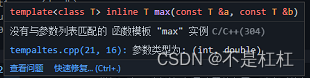函数模板重载

#include <iostream>
template <typename T> //模板参数由关键字typename（也可以使用class） 定义 定义一个函数模板 返回两个数中数值大的那一个
inline T max(const T &a,const T &b)//函数有两个参数 ，类型为定义
{
return a>b?a:b;
}

#include <iostream>
template <typename T>

inline T max(const T &a,const T &b,const T &c)
{
T temp;
if(a<b)
{
temp = b;
}
else
{
temp = a;
}
return temp >c?temp :c;

}

inline int max(const int &a,const int &b)
{
return a>b?a:b;
}

template <typename T,typename T1=double>
inline T max(const T &a,const T1 &b)
{
std::cout<<"template <typename T,typename T1=double> "<<std::endl;
return a>b?a:b;
}

int main()
{
max(1,2,3);     // max(const T &a,const T &b,const T &c) max<int>
max(1.0,2.3);     //max(const T &a,const T &b) max<double>
max('a','b');    //max(const T &a,const T &b)  max<char>
max(1,2);    //inline int max(const int &a,const int &b)
max<>(1,2);    //max(const T &a,const T &b) max<int>
max<int>(1,2);    //max(const T &a,const T &b) max<int>
max('a',2);    //inline int max(const int &a,const int &b)
return 0;
}


# 类模板

与函数模板类似，类也可以通过参数泛化，从而可以构建出一族不同的类实例（对象）

类模板实参可以是某一类型或常量（仅限int或enum）

示例

#include<iostream>
#include<vector>
/*********************************************************
*T可以是任意类型
*模板实参也可以是一个int或enum类型的常量 size_t实际是int类型
*n是编译时定义的常量
*n可以有默认值
*size_t类型的成员变量可以用n初始化
***********************************************************/
const std::size_t DefaultStackSize = 1024;
template <typename T,std::size_t n = DefaultStackSize>
class Stack
{
public:
void Push(const T &element);
int Pop(T &element);
//int Top(T &element) const;
private:
std::vector<T> m_Members;
std::size_t m_mMaxSize = n;
};

template <typename T,std::size_t nMaxSize>
void Stack<T,nMaxSize>::Push(const T &element)
{
if(m_mMaxSize <= m_Members.size())
{
return;
}
m_Members.push_back(element);
}

template <typename T,std::size_t nMaxSize>
int Stack<T,nMaxSize>::Pop(T& element)
{
if(m_Members.empty())
{
return -1;
}
element = m_Members.back();
m_Members.pop_back();
return 0;
}

int main()
{
Stack<int> stack;
Stack<int,100> stack1;
for(int i =0;i<100;i++)
{
stack.Push(i);
}
int i;
stack.Pop(i);
std::cout<<i<<std::endl;
stack.Pop(i);
std::cout<<i<<std::endl;
return 0;
}

## 类模板特化

允许对一个类模板的某些模板参数类型做特化

### 特化的作用和好处

对于某种特殊的类型，可能可以做些特别的优化或提供不同的实现

避免在实例化的时候引起一些可能不好的行为

特化一个类模板的时候也意味着需要特化其所有参数化的成员函数

示例：

#include<iostream>
#include<vector>
/*********************************************************
*T可以是任意类型
*模板实参也可以是一个int或enum类型的常量 size_t实际是int类型
*n是编译时定义的常量
*n可以有默认值
*size_t类型的成员变量可以用n初始化
***********************************************************/
const std::size_t DefaultStackSize = 1024;
template <typename T,std::size_t n = DefaultStackSize>
class Stack
{
public:
void Push(const T &element);
int Pop(T &element);
//int Top(T &element) const;
private:
std::vector<T> m_Members;
std::size_t m_mMaxSize = n;
};

template <typename T,std::size_t nMaxSize>
void Stack<T,nMaxSize>::Push(const T &element)
{
if(m_mMaxSize <= m_Members.size())
{
return;
}
m_Members.push_back(element);
}

template <typename T,std::size_t nMaxSize>
int Stack<T,nMaxSize>::Pop(T& element)
{
if(m_Members.empty())
{
return -1;
}
element = m_Members.back();
m_Members.pop_back();
return 0;
}
template <>
class Stack<std::string>
{
public:
void Push(const T &element);
int Pop(T &element);
//int Top(T &element) const;
private:
std::list<string> m_Members;
std::size_t m_mMaxSize;
};

int main()
{
Stack<int> stack;
Stack<int,100> stack1;
for(int i =0;i<100;i++)
{
stack.Push(i);
}
int i;
stack.Pop(i);
std::cout<<i<<std::endl;
stack.Pop(i);
std::cout<<i<<std::endl;
return 0;
}

类模板也可以偏特化

template <typename T1,typename T2>
class MyClass
{
};

//偏特化
template <typename T>
class MyClass<T,T>
{};

template <typename T1,typename T2>
class MyClass<T1*,T2*>
{};

template <typename T>
class MyClass<T,int>
{};



展开全文开发语言
• C++模板元编程实战：一个深度学习框架的初步实现》以一个深度学习框架的初步实现为例，讨论如何在一个相对较大的项目中深入应用元编程，为系统性能优化提供更多的可能。
• 说到C++模板，这个已经不是什么新东西了，自己在实际开发中也用过；对于C++模板特化和偏特化，对于别人来说，已经不是什么新东西了，但是对于我来说，的确是我的盲区，那天在群里讨论这个问题，自己对于这部分确实...
• C++模板元编程.pdf 个人收集电子书，仅用学习使用，不可用于商业用途，如有版权问题，请联系删除！
• 主要介绍了C++模板的详细介绍，欢迎大家下载学习啦啦啦啦
• c++，类、模板、链表的简单使用，包括入链表、出链表等等
• 所谓元编程就是编写直接生成或操纵程序的程序,C++ 模板C++ 语言提供了元编程的能力,模板使 C++ 编程变得异常灵活,能实现很多高级动态语言才有的特性
• ## C++模板函数

千次阅读 2021-10-10 15:30:18
C++提供了模板(template)编程的概念。所谓模板，实际上是建立一个通用函数或类，其类内部的类型和函数的形参类型不具体指定，用一个虚拟的类型来代表。这种通用的方式称为模板模板是泛型编程的基础,泛型编程即以一...

## 1.模板函数

### 1.模板函数的声明与定义

C++提供了模板(template)编程的概念。所谓模板，实际上是建立一个通用函数或类，其类内部的类型和函数的形参类型不具体指定，用一个虚拟的类型来代表。这种通用的方式称为模板。模板是泛型编程的基础,泛型编程即以一种独立于任何特定类型的方式编写代码。

简单来说，就是我们提供一个抽象的函数，并不具体指定其中数据的类型，而是某个虚拟类型代替。只提供基本的功能。其具体的数据类型，只在其被调用时视具体情况实例化。

这么说可能还是很抽象，举个例子就好理解了。

#include <iostream>
#include <stdio.h>

using namespace std;

template <typename T1,typename T2>             //模板函数声明与定义
T2 test(T1 tmp, T2 tmp1) {

T2 tmp2 = tmp + tmp1;

return tmp2;
}

int main(void) {

cout << "test(10, 5)=" << test(10, 5) << endl;     //调用模板函数，模板函数通过传入的参数自动推导未实例化的类型
cout << "test(5,'A')=" << test(5,'A') << endl;
cout << "test(10.5, 5.5) =" << test(10.5, 5.5) << endl;

system("pause");

return 0;
}


输出结果为：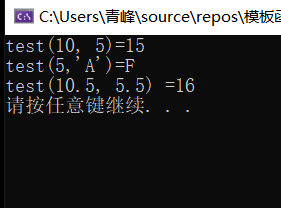函数模板的声明通过关键字template与typename 实现。其中，template告知编译器这是函数模板的声明，typename用来声明虚拟类型。比如你要声明一个模板函数，里面需要两个不同的变量，那么你就需要通过typename声明两个不同的虚拟类型T1，T2。

声明好后，你就可以在函数定义中使用虚拟类型来定义变量，但是要注意，用同一个虚拟类型定义的变量就只能是同种类型，比如用T1定义的变量只能是同种变量，可以是int,也可以是char。这取决于其实例化时被实例化为哪种类型。

### 2.模板函数的调用

#### 显式类型调用

可以显式的调用模板函数，即在调用时人为地指明虚拟类型的具体类型。

#include <iostream>
#include <stdio.h>

using namespace std;

template <typename T1,typename T2>             //模板函数声明与定义
T2 test(T1 tmp, T2 tmp1) {

T2 tmp2 = tmp + tmp1;

return tmp2;
}

int main(void) {

cout << "test(5,'A')=" << test<int,char>(5, 'A') << endl;          //<int,char>显式的指明模板的类型

system("pause");

return 0;
}


#### 自动推导

即不指明具体的数据类型，而让编译器根据传入的数据自动推导出数据类型。

#include <iostream>
#include <stdio.h>

using namespace std;

template <typename T1,typename T2>             //模板函数声明与定义
T2 test(T1 tmp, T2 tmp1) {

T2 tmp2 = tmp + tmp1;

return tmp2;
}

int main(void) {

cout << "test(5,'A')=" << test(5, 'A') << endl;          //自动推导数据类型

system("pause");

return 0;
}


### 3.模板函数的底层实现

模板函数在被实例化后，那么它就会被具体的函数所取代。举个例子，某一版代码有一个模板函数，其被调用后虚拟类型被自动推导为int型。那么在编译后就会生成实例化为int型的函数（即所有虚拟类型被int取代的普通函数），而不会调用模板函数，也就是在调用时，模板函数会被实例化为普通函数的函数所取代。

由此我们或许可以知道，无论是自动推导，还是显式的指定类型。都不允许出现不能被编译器知道的虚拟类型。更清楚的说，就是使用自动推导方式推导模板函数类型时必须要让编译器能推导出具体的类型。

看个不能被编译器推导出的例子。

错误代码

#include <iostream>
#include <stdio.h>

using namespace std;

template <typename T1,typename T2>             //模板函数声明与定义
T1 test(T1 tmp, T1 tmp1) {

T2 tmp2;               //T2无法被推导出具体的类型

return (tmp + tmp1);
}

int main(void) {

cout << "test(5, 6)=" << test(5, 6) << endl;

system("pause");

return 0;
}


### 4.模板函数与函数重载

熟悉函数重载的人应该会好奇，如果既有模板函数又有同名的普通函数，而且参数列表的参数个数是一样的，那么在主函数中调用同名函数，编译器具体会调用哪一个呢？

下面看一个例子：

#include <iostream>
#include <stdio.h>

using namespace std;

template <typename T1,typename T2>             //模板函数声明与定义
T1 test(T1 tmp, T2 tmp1) {

cout << "调用模板函数！" << endl;

return (tmp + tmp1);
}

int test(int tmp, int tmp1) {                  //重载的普通函数

cout << "调用普通函数！" << endl;

return 0;
}

int main(void) {

char tmp = 'c';
int tmp1 = 0;
int a = 5;

cout << "test(5,'c')=" << test(a, tmp) << endl;
cout << "test(5,0)=" << test(a, tmp1) << endl;

system("pause");

return 0;
}


结果为：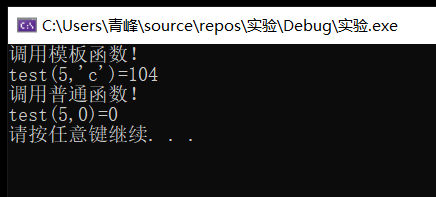普通函数的两个参数都是int型，在第一次调用test时第二个参数使用的是char型，调用的是模板函数，第二次使用的是int型，调用的是普通函数。

这是为什么呢？理论上来说，模板函数两个都能匹配，使用。而普通函数也能匹配这两次调用的参数（在C语言中，char型变量是可以作为int型参数使用的）。

这是因为模板函数可以自动推导类型，在第一次调用中，两个类型分别被推导为int型与char型。而普通函数是两个int型，虽然也能使用传入的参数，但模板函数明显能更好的匹配参数列表。

也就是说，如果模板函数实例化后的类型能更好的匹配参数列表的话就使用模板函数。

那么当这两个函数都能完全匹配参数列表的时候呢？通过第二次test的调用结果不难发现，这时候，编译器会调用普通函数。

如果一定要使用模板函数，可以使用<>显式的指定使用模板函数。看下面的例子。

#include <iostream>
#include <stdio.h>

using namespace std;

template <typename T1,typename T2>             //模板函数声明与定义
T1 test(T1 tmp, T2 tmp1) {

cout << "调用模板函数！" << endl;

return (tmp + tmp1);
}

int test(int tmp, int tmp1) {                  //重载的普通函数

cout << "调用普通函数！" << endl;

return 0;
}

int main(void) {

char tmp = 'c';
int tmp1 = 0;
int a = 5;

cout << "test(5,'A')=" << test(a, tmp) << endl;
cout << "test<>(5,0)=" << test<>(a, tmp1) << endl;       //使用<>显式的调用模板函数

system("pause");

return 0;
}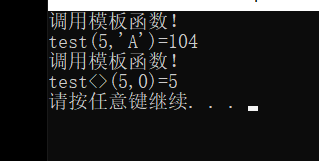## 2.下文

下篇文章我们介绍类模板。

链接：

C++类模板

展开全文开发语言 后端
• 介绍C++编程语言中，模板相关的理论介绍，原理设计，代码设计技巧等
• 　答：class用于定义类，在模板引入c++后，初定义模板的方法为：template，这里class关键字表明T是一个类型，后来为了避免class在这两个地方的使用可能给人带来混淆，所以引入了typename这个关键字，它的作用同...
• 深入学习与实践c++模板，值得借鉴与收藏，不懂的看了可以入门，懂的看了可以了解更多，学得更多。
• 侯捷老师力作,详细讲解了C++模板知识,C++高级开发必备资料,偶然所得,为了保存此资料,上传至CSDN.目前资源分设定至少为1,感觉不利于大家共享资料
• ## C++模板函数

千次阅读 2022-01-21 19:11:24
C++模板函数

# 前言

今天在学习算法的时候，接触到了C++模板函数的概念，顺便练习整理一下知识点

# 模板函数

模板函数声明语句: template <typename T> 函数参数中具体的数据类型可由统一模板T代替

(模板名习惯上设置为T，可自定义)

# 案例

定义一个选择排序的模板，分别对不同类型的数组进行排序并输出

（其中整型数组为随机数组成的数组，省去手动输入测试）

随机生成数详解 C++中引入<ctime>库函数，这一点与C不同，其它的原理一致。

代码块

//定义模板函数
template <typename T>
void SelectSort(T a[],int n){
for(int i=0;i<n;i++){
int min_index=i;
for(int j=i+1;j<n;j++)
if(a[j]<a[min_index])
min_index=j;
swap(a[i],a[min_index]);
}
}

测试

#include<iostream>
#include<algorithm>
#include<ctime>
using namespace std;
/*
1.自动生成一个随机数组
2.对其进行选择排序
3.使用模板函数进行不同类型数据的排序
如：整型，浮点型，字符型，结构体类型（自定义）
*/
//定义模板函数
template <typename T>
void SelectSort(T a[],int n){
for(int i=0;i<n;i++){
int min_index=i;
for(int j=i+1;j<n;j++)
if(a[j]<a[min_index])
min_index=j;
swap(a[i],a[min_index]);
}
}

template <typename P>
void PrintArray(P a[],int n){
for(int i=0;i<n;i++){
cout<<a[i]<<" ";
}
cout<<endl;
}
//随机数组
int* RandomArray(int n,int rangeL,int rangeR){
int *arr=new int[n];//创建一个大小为n的数组
srand(time(NULL));//以时间为"种子"产生随机数
for(int i=0;i<n;i++){
arr[i]=rand()%(rangeR-rangeL+1)+rangeL;//生成指定区间[rangeL,rangeR]里的数
}
return arr;
}

int main(){
float b={0.5,2.7,1.5,15.8,10.2};
char c={'e','a','c','d','b'};
int n;
cout<<"请输入数据规模n：";cin>>n;
int* a=RandomArray(n,1,100);//生成[1,100]内的随机数组成的数组
cout<<"整型排序：";SelectSort(a,n); PrintArray(a,n);//整型
cout<<"浮点型排序：";SelectSort(b,5); PrintArray(b,5);//浮点型
cout<<"字符型排序：";SelectSort(c,5); PrintArray(c,5);//字符型
return 0;
}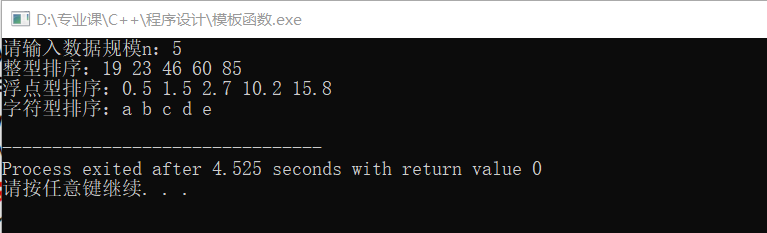对于模板函数，我只是简略整理下（知道有这个东西，会用就行），更具体的知识点与用法还得看这位大佬的博客。C++模板函数详解

展开全文• 深入实践C++模板编程-高清-2013年6月,分享给所有需要的人!
• 函数模板嵌套 template <class _Ty1,class _Ty2> class MM { public: MM(_Ty1 one, _Ty2 two) :one(one), two(two) {} friend ostream& operator<<(ostream& out, const MM& mm) { ...
• 所谓元编程就是编写直接生成或操纵程序的程序，C++ 模板C++ 语言提供了元编程的能力，模板使 C++ 编程变得异常灵活，能实现很多高级动态语言才有的特性（语法上可能比较丑陋，一些历史原因见下文）。普通用户对 ...
• 当类模板碰到继承时：注意以下几点： 当子类继承的父类是一个类模板时，子类在声明的时候，要指定出父类中T的类型。明确T的类型。 如果不指定，编译器无法给子类分配内存。 如果想灵活指定父类中T的类型，子类也...
• ## C++模板类详解及注意事项

万次阅读 多人点赞 2020-08-16 09:11:55
C++模板 C++语言引入模板技术，它使用参数化的类型创建相应的函数和类，分别称之为函数模板和类模板 函数模板： 可以用来创建一个通用功能的函数，以支持多种不同形参，进一步简化重载函数的函数体 请参考:函数...编程语言
• 前言：大家好，今天给大家分享一篇关于 c++ 模板总结概述.模板（Template）指 C++ 程序设计设计语言中采用类型作为参数的程序设计，支持通用程序设计。C++ 的标准库提供许多有用的函数大多结合了模板的观念，如 STL ......

# c++模板c++ 订阅